## Calculation of Information and Complexity —Concepts, Requirements and Limits

### Frank Wolf

This article has been extracted from the author's thesis. It gives a brief overview on the calculation of some measures of information and complexity from a practical point of view. Please refer to the literature as e. g. listed in the thesis for further details and theoretical backgrounds.

1. Alphabets and Symbol Sequences
2. Distributions of Words
3. Notation
4. Measures of Information
4.1 Shannon Entropy
4.2 Metric Entropy
4.3 Rényi Entropy
4.4 Mean Information Gain
4.5 Mean Mutual Information
5. Measures of Complexity
5.1 Effective Measure Complexity
5.2 Fluctuation Complexity
5.3 Rényi Complexity
6. Required Amount of Data and Maximum Word Length
6.1 Background
6.2 Calculator
7. Literature

### 1. Alphabets and Symbol Sequences

The calculation of information and complexity is restricted to one-dimensional equidistant time series, here. The value range of a time series is restricted to the symbols of a small alphabet. Thus, we are talking about symbol sequences instead of time series. Examples of alphabets are:

• binary, two symbols: The smallest alphabet, e. g. 0 and 1 (computer code)
• quaternary, four symbols: A DNA sequence can be denoted as a symbol sequence based on the symbols A, C, G, T, because each of the cords of the double-helix consists of the bases Adenin, Cytosin, Guanin and Thymin.
• Of course, a text from a book, newspaper, etc. can be regarded as symbol sequence as well. Then the alphabet is the natural alphabet of the respective language including special symbols and digits.

If a time series is not a symbol sequence, then it can be transformed into a symbol sequence by e. g. partitioning the value range. Then, certain subranges of the value range can be mapped to certain symbols. A prefered partition is e. g. the mapping of all values below the median of the time series to symbol '0' and all values above to symbol '1'. Thus, a binary symbol sequence reveals with about an equal number of zeros and ones.

### 2. Distributions of Words

The calculation of information and complexity bases on distributions of subsequences of the symbol sequence – the so-called words. At first, you have to commit to a word length L. The words of length L are denoted as L-words. Now, determine the relative frequency pL,i of each of the occuring L-words. Some measures require transition frequencies between words in addition.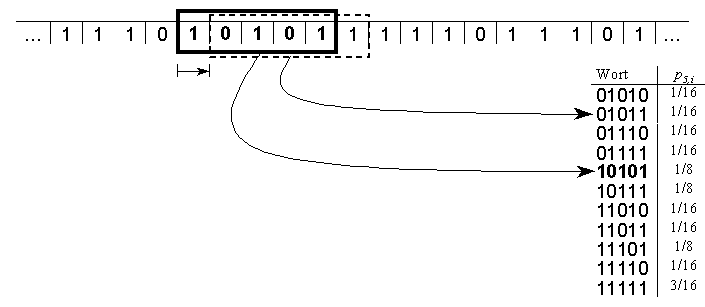In the figure, you see a binary symbol sequence. A window of length 5 is sliding over the sequence, each time shifted by one symbol. Thereby, a list of all occuring words with their relative frequencies is created.

### 3. Notation

Note: The measure of information and complexity are defined on probability distributions. But these are unknown for practical data like e. g. measurement data, texts, DNA, etc.. In that case, the probabilities have to be approximated by relative frequencies while calculating the measures. Be aware that we do not distinguish between relative frequencies and probabilities in the notation of this article.

The following terms and symbols are used in the formula below:
 L word length i index of the i-th L-word, L-word i λ number of symbols in alphabet, alphabet size n number of different L-words, maximum λL pi, pL,i probability of occurence for i-th L-word pL,ij probability for simultanuous occurence of the i-th and j-th L-word, where word j is a word that follows word i overlapping. Thus, the two words share the same L-1 symbols. pi, pL,i→j Conditional probability for the occurence of the j-th L-word, if the i-th L-word has been observed before

### 4. Measures of Information

#### 4.1 Shannon Entropy

While investigating the transmission of messages in telephone lines, Shannon (1948) defined the historically first measure of information - the Shannon Entropie: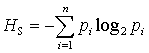(1)
Shannon's notion of information does not measure the semantical content or the meaning of a message. It measures the message's unpredictability (randomness, disorder). A message is the more informative, the more uncertain we are according to its syntactical content. The Shannon entropy is the classical measure of information. The following measures of information are a kind of refinement of this measure. The name "Entropy" roots in the analoguous meaning of the formula in thermodynamics.

#### 4.2 Metric Entropy

The Shannon entropy increases linearly with increasing word length. Normalization with the word length yields the same notion of information, but independently of the word length. This measure is called Metric Entropy: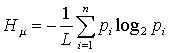(2)
The metric entropy vanishes for constant sequences, increases monotonically when the sequence's disorder raises and reaches its maximum at 1 for equally distributed random sequences.

#### 4.3 Rényi Entropy

Rényi (1960 u. 1961) proposed a generalization of the Shannon entropy - the Rényi Entropy of the order α: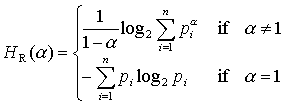(3)
The parameter α allows to weight seldom or frequent words differently. High probabilities are strengthened compared to low probabilities for α > 1. If α < 1, then the lower probabilities are strengthened.

#### 4.4 Mean Information Gain

The knowledge of a symbol that follows an L-word contributes to the local information. The average gain of information leads to the definition of another important measure of information: The mean information gain.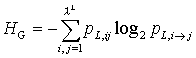(4)
This formula is equivalent to a difference of Shannon entropies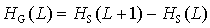(5)
The compact formula requires less computation time and is numerically more stable.
This notion of information can easily be visualized using the example of one word. If one observes the sequence of letters “info” within this text, then the following letter will be an “r” for sure. The knowledge of this following letter does not contribute to the information. But, if we have observed the sequence “the_”, then it is really hard to predict the next letter. Any letter is possible, because it is the first letter of some word of the english language. Here, the knowledge of the next letter is a real gain of information. The mean information gain HG tells us, how much additional information we gain in average for the whole symbol sequence by knowing the next symbol.

#### 4.5 Mean Mutual Information

The mean mutual information measures how much information is in the dependency (correlation) of two successive words. It is defined after Wackerbauer et al. (1994) as: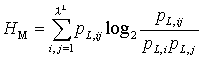(6)
This formula is theoretically equivalent to a difference of Shannon entropies: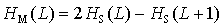(7)
The compact formula requires less computation time. Additionally, there are differences in the precision as shown by the calculator.

### 5. Measure of Complexity

#### 5.1 Effective Measure Complexity

The minimum total amount of information that has to be stored at any time for an optimal prediction of the next symbol is measured after Grassberger (1986, S. 920) by the Effective Measure Complexity. This measure is defined as a limit value and can be approximated by the formula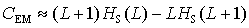(8)
Faster to calculate and numerically more stable is the equivalent formula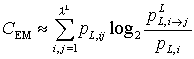(9)

#### 5.2 Fluctuation Complexity

While shifting from L-word i to L-word j the newly observed symbol contributes to a gain of information. This lead to the definition of the mean information gain. At the same time there is a loss of information, because we forget the first symbol of word i. In average for the whole symbol sequence, information gain and loss eliminate each other. The fluctuation between these two values is measured by the fluctuation complexity after Bates & Shepard (1993).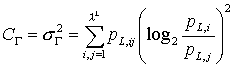(10)
In the example for the information gain, we observed the 4-word “info”, which has been followed by the 4-word “nfor”. The information gain by knowing the “r” is zero within this text, whereas the information loss of forgetting the “i” is higher, because “nfor” occurs in the words “Unfortunately” and “Information” with a capital “I” (at the begin of a sentence or in headings). The more this balance of information gain and loss is fluctuating in the investigated text, the more complex is the text in the sense of the fluctuation complexity.

#### 5.3 Rényi Complexity

According to an oral recommendation of Prof. Dr. Jürgen Kurths a measure of complexity can be defined by regarding the differences of Rényi entropies of conjugated orders. This leads to an own definition of a measure of complexity. Let the Rényi Complexity CR(α) of the order α > 1 of a distribution of L-words be: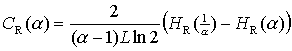(11)
The scaling factor yields independency of the word length and eliminates the zero for α → 1. As shown in investigations, CR is specific in the sense of a measure of complexity only for α ≈ 1. Thus, let the Rényi Complexity be defined as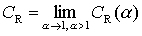(12)
As found out, this measure is sufficient numerically stable approximated by CR(1.0001). The Rényi complexity judges dynamics in a qualitatively similar way as the fluctuation complexity does. But, the Rényi complexity revealed as the more stable alternative.

## 6. Required amount of data and maximum word length

### 6.1 Background

As already mentioned in the notation section, measures of information and complexity are defined on probability distributions (of the L-words). But, these are unknown for practical data and have to be estimated from the relative frequencies. Obviously, these estimates may be impossible for large word lengths compared to the available amount of data. This leads to huge errors in the measures. For N data points there are NL+1 words. Theoretially there are a maximum of λL different words. Thus, not all theoretically possible words may be observable (sufficiently frequent) even if the word length is comparable small. Unfortunately, the description of the dynamic data structure usually requires higher word lengths. This fixes the word length to the maximum size that still allows a sufficient precise calculation of a measure.

Such a value cannot be determined for all possible distributions of words. But an estimate for the worst case is possible. This is the case of equally distributed random sequences, because here all words are possible and the data stock is exhausted at first compared to other distributions. In that case the probability to observe a word one time, two times, three times etc. follows a binomial distribution. So, for each measure of information or complexity an expected value depending on the amount of data, word length and alphabet size can be calculated. The respecitve formula have been extracted in the thesis. The theoretical value of the measures in this case is also known. Thus, a condition of tolerance for the deviation (relative precision) of the expected value compared to the theoretical value can be stated. For a given maximum relative error (minimum precision) the required amount of data can be determined for each alphabet size and word length. This requires immense computation resources, because the unequations cannot be solved analytically. Such calculations have been performed within the thesis for some relevant parameter combinations. I. e. for 1 % and 2 % relative precision, binary, ternary and quaternary alphabets and all word lengths until more than 1000000 data points become required. The results are presented here with help of the JavaScript calculator.

### 6.2 Calculator

The JavaScript calculator helps you to find out, which word length is possible for a given amount of data, or which data amount is required for a given word length. The calculator considers the worst (most data consuming) case. Therefore you should be aware of the following:

• Mind the recommendations of the calculator, if you are investigating high-information data, like e. g. DNA sequences, precipitation, random sequences, irrational numbers.
• Mind the recommendations of the calculator, too, if you are comparing different data sets — including high-information data.
• You may choose bigger word lengths and alphabets with less amounts of data, if you are sure investigating medium- or low-information data, only. This is the case for texts, books, etc. Then you are encouraged to do your own data amount / word length investigations.

Precision:
1 %
5 %
 Alphabet size: 2, binary 3, ternary 4, quaternary
 Minimum required amount of data for word length Maximum word length for data points

The measures in the calculator refer to the following formula: Shannon entropy: (1), (2) and (3) with α ≈ 1; Information gain: (4) and (5); Mutual information: diff. (7), comp. (6); Effective measure complexity: (8) and (9); Fluctuation complexity: (10); Rényi complexity: (11) with α = 1.0001.

## 7. Literature

Bates, JE; Shepard, HK (1993): Measuring complexity using information fluctuation. Physics Letters A, 172: 416–425.
Grassberger, P (1986): Toward a Quantitative Theory of Self-Generated Complexity. International Journal of Theoretical Physics, 25 (9): 907–938.
Rényi, A (1960): Some Fundamental Questions of Information Theory. In: Pál Turán (Hrsg.): Selected Papers of Alfréd Rényi. Vol. 2: 1956 — 1961. Budapest: Akadémiai Kiadó, 1976: 526–552.
Rényi, A (1961): On measures of entropy and information. In: Pál Turán (Hrsg.): Selected Papers of Alfréd Rényi. Vol. 2: 1956 — 1961. Budapest: Akadémiai Kiadó, 1976: 565–580.
Shannon, CE (1948): A Mathematical Theory of Communication. Bell System Technical Journal, 27: 379–423.
Wackerbauer, R; Witt, A; Atmanspacher, H; Kurths, J; Scheingraber, H (1994): A Comparative Classification of Complexity Measures. Chaos, Solitons & Fractals, 4: 133–173.
A more complete list of literature is provided in the thesis.
 Last change: June, 10th 2001 Accesses since February, 21th 2001: